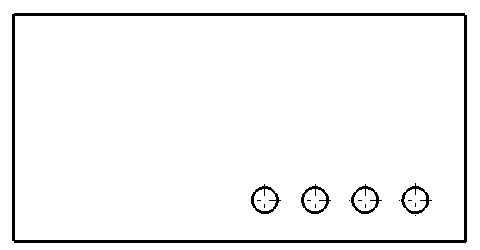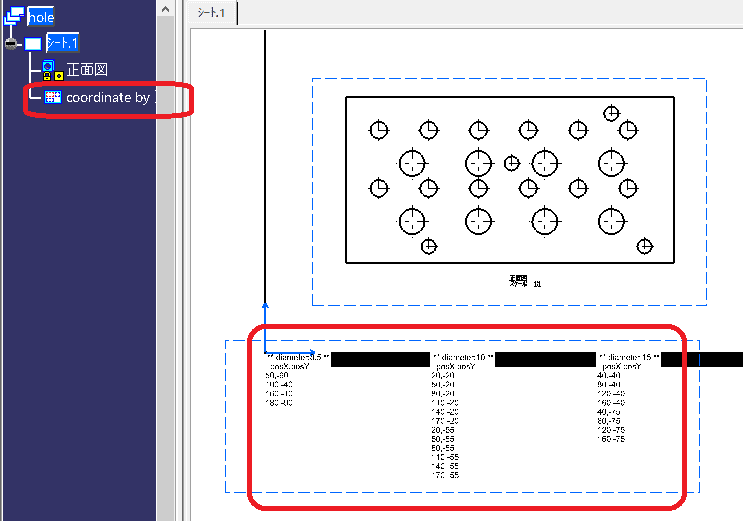×
INTELLIGENT WORK FORUMS
FOR ENGINEERING PROFESSIONALS

Are you an
Engineering professional?
Join Eng-Tips Forums!
• Talk With Other Members
• Be Notified Of Responses
• Keyword Search
Favorite Forums
• Automated Signatures
• Best Of All, It's Free!

*Eng-Tips's functionality depends on members receiving e-mail. By joining you are opting in to receive e-mail.

#### Posting Guidelines

Promoting, selling, recruiting, coursework and thesis posting is forbidden.

# Find the circle

## Find the circle

(OP)
I have a projection drawing from 3d, i need to find holes in circle in 2d drawing bane with macro but failed. Can anyone help me see where the error is?
Sub CATMain()

Dim drawingDocument1 As DrawingDocument
Set drawingDocument1 = CATIA.ActiveDocument

Dim SelectionsAll As Selection
Set Selection = drawingDocument1.Selection
MsgBox Selection.Count2
For i = 1 To Selection.Count2
Set RefView1 = Selection.Item(i).Value
Set Element = RefView1.GeometricElements
'Set Element = refView.GeometricElements.GetCurvature RefView
MsgBox TypeName(Element)
Select Case TypeName(Element)
Case "Axis2D":
MsgBox "Axis"
Case "Line2D":
MsgBox "line"
Case "Circle2D":
MsgBox "Circle"
End Select
Next
MsgBox "finish"
End Sub### RE: Find the circle

Do you mean to count circles appearing in 2D sheet? If you want to find anything its not right code, firstly i recommend using method selectelement3 with triggering palette instead of preselection.

Regards,
M

### RE: Find the circle

Geometry generated from 3D is not exposed to Automation.

### RE: Find the circle

(OP)
Little Cthulhu do you have any command to find them? tôi đang muốn tạo một macro như lệnh " hole dimension table" của catia

### RE: Find the circle

No, you can't find generated geometry by type (circle, line etc.)

### RE: Find the circle

(OP)
is there a way to select the circle?

### RE: Find the circle

hoangthe.

You can find the circle by Isolating the link.
This is not a very pretty method, but here is an example of temporarily copying and pasting a view, Isolating it, and then deleting it once the necessary information is retrieved.
A new view is created near the origin, and the result is text for each diameter.

#### CODE --> vba

'vba

Option Explicit

Sub CATMain()

Dim dDoc As DrawingDocument
Set dDoc = CATIA.ActiveDocument

Dim msg As String
msg = "Select the view from which to extract the coordinates of the hole / ESC = Cancel"

Dim selElement As SelectedElement
Set selElement = select_element( _
msg, _
Array("DrawingView") _
)
If selElement Is Nothing Then Exit Sub

Dim view As DrawingView
Set view = selElement.value

Dim cloneView As DrawingView
Set cloneView = get_clone_view(view)
'
Dim circles As Collection
Set circles = get_circles(cloneView)
If circles Is Nothing Then
remove_entity cloneView
Exit Sub
End If

remove_entity cloneView

Call dump_circle_info( _
dDoc.sheets.ActiveSheet, _
"coordinate by " & view.name, _
)

MsgBox "Done"

End Sub

Private Function dump_circle_info( _
ByVal sheet As DrawingSheet, _
ByVal viewName As String, _
ByVal dict As Object)

Dim numericKeys As Variant
numericKeys = quick_sort(get_numeric_keys(dict))

Dim coordinateView As DrawingView
Set coordinateView = create_view( _
sheet, _
viewName _
)

Dim a
Dim txt As DrawingText
Dim idx As Long
Dim coordinates As Collection
Dim coord As Collection
Dim infoLst As Collection
For idx = 0 To UBound(numericKeys)
Set infoLst = New Collection
infoLst.Add " ** diameter:" & numericKeys(idx) & " **"
Set coordinates = dict.Item(Trim(Str(numericKeys(idx))))
For Each coord In coordinates
Next

Join(col2ary(infoLst), vbCrLf), _
idx * 100, _
0 _
)
Next

End Function

Private Function get_numeric_keys( _
ByVal dict As Object) _
As Variant

Dim numericKeys() As Variant
ReDim numericKeys(dict.count - 1)

Dim ary As Variant
ary = dict.keys

Dim i As Long
For i = 0 To dict.count - 1
numericKeys(i) = CDbl(ary(i))
Next

get_numeric_keys = numericKeys

End Function

Private Function create_view( _
ByVal sheet As DrawingSheet, _
Optional ByVal name As String = vbNullString) _
As DrawingView

If name = vbNullString Then
name = "AutomaticNaming"
End If

End Function

ByVal circles As Collection, _
Optional ByVal roundTolerance As Long = 3) _
As Object 'dict

Dim group As Object
Set group = init_dict()

Dim values As Collection

Dim center(1) As Variant
Dim dia As Double
Dim key As String
Dim c As Variant ' Circle2D
For Each c In circles
dia = Round(c.radius * 2, roundTolerance)
Call c.GetCenter(center)
key = Trim(Str(dia))

If group.Exists(key) Then
Else
Set values = New Collection
End If
Next

End Function

Private Function col2ary( _
ByVal col As Collection) _
As Variant

Dim ary() As Variant
ReDim ary(col.count - 1)

Dim i As Long
For i = 1 To col.count
ary(i - 1) = col.Item(i)
Next

col2ary = ary

End Function

Private Function ary2col( _
ByVal ary As Variant) _
As Collection

Dim col As Collection
Set col = New Collection

Dim i As Long
For i = 0 To UBound(ary)
Next

Set ary2col = col

End Function

Private Sub remove_entity( _
entity As AnyObject)

Dim sel As Selection
Set sel = CATIA.ActiveDocument.Selection

CATIA.HSOSynchronized = False

With sel
.Clear
.Delete
End With

CATIA.HSOSynchronized = True

End Sub

Private Function get_circles( _
ByVal view As DrawingView) _
As Collection

Set get_circles = Nothing

Dim sheet As DrawingSheet
Set sheet = get_parent_of_T(view, "DrawingSheet")

Dim sel As Selection
Set sel = CATIA.ActiveDocument.Selection

CATIA.HSOSynchronized = False

With sel
.Clear
.Search "CATDrwSearch.2DCircle,sel"
End With

If sel.Count2 < 1 Then
CATIA.HSOSynchronized = True
Exit Function
End If

Dim circles As Collection
Set circles = New Collection

Dim i As Long
For i = 1 To sel.Count2
Next

sel.Clear

CATIA.HSOSynchronized = True

Set get_circles = circles

End Function

Private Function get_clone_view( _
ByVal view As DrawingView) _
As DrawingView

Dim sheet As DrawingSheet
Set sheet = get_parent_of_T(view, "DrawingSheet")

Dim sel As Selection
Set sel = CATIA.ActiveDocument.Selection

CATIA.HSOSynchronized = False

With sel
.Clear
.Copy
.Clear

.Paste
End With

Dim tempView As DrawingView
Set tempView = sel.Item2(1).value

sel.Clear

CATIA.HSOSynchronized = True

tempView.LockStatus = False
tempView.Isolate

Set get_clone_view = tempView

End Function

Private Function get_parent_of_T( _
ByVal aoj As AnyObject, _
ByVal t As String) _
As AnyObject

Dim aojName As String
Dim parentName As String

On Error Resume Next
aojName = aoj.name
parentName = aoj.Parent.name
On Error GoTo 0

If TypeName(aoj) = TypeName(aoj.Parent) And _
aojName = parentName Then
Set get_parent_of_T = Nothing
Exit Function
End If
If TypeName(aoj) = t Then
Set get_parent_of_T = aoj
Else
Set get_parent_of_T = get_parent_of_T(aoj.Parent, t)
End If

End Function

Private Function init_dict( _
Optional CompareMode As Long = vbBinaryCompare) _
As Object

Dim dict As Object
Set dict = CreateObject("Scripting.Dictionary")
dict.CompareMode = CompareMode
Set init_dict = dict

End Function

Private Function quick_sort( _
ByVal ary As Variant) As Variant

If IsEmpty(ary) Then
quick_sort = Empty
Exit Function
End If

Dim stack As Object
Set stack = init_dict()

Dim leftIdx As Long
Dim rightIdx As Long
Dim pivot As Variant
Dim tPivot(2) As Variant
Dim temp As Variant

Dim i As Long
Dim j As Long
Do While stack.count > 0

leftIdx = stack(stack.count - 1)
rightIdx = stack(stack.count)
stack.Remove stack.count
stack.Remove stack.count

If leftIdx < rightIdx Then

pivot = ary((leftIdx + rightIdx) / 2)

i = leftIdx
j = rightIdx

Do While i <= j

Do While ary(i) < pivot
i = i + 1
Loop

Do While ary(j) > pivot
j = j - 1
Loop

If i <= j Then
temp = ary(i)
ary(i) = ary(j)
ary(j) = temp

i = i + 1
j = j - 1
End If

Loop

If rightIdx - i >= 0 Then
If rightIdx - i <= 10 Then
insertion_sort ary, i, rightIdx
Else
End If
End If

If j - leftIdx >= 0 Then
If j * leftIdx <= 10 Then
insertion_sort ary, leftIdx, j
Else
End If
End If
End If

Loop

quick_sort = ary
End Function

Private Function insertion_sort( _
ary As Variant, _
minIdx As Long, _
maxIdx As Long)

Dim i As Long, j As Long
Dim temp As Variant
j = 1
For j = minIdx To maxIdx
i = j - 1
Do While i >= 0
If ary(i + 1) < ary(i) Then
temp = ary(i + 1)
ary(i + 1) = ary(i)
ary(i) = temp
Else
Exit Do
End If
i = i - 1
Loop
Next

insertion_sort = ary
End Function

Private Function select_element( _
ByVal msg As String, _
ByVal filter As Variant _
) As SelectedElement

Dim sel As Variant
Set sel = CATIA.ActiveDocument.Selection

sel.Clear
Select Case sel.SelectElement2(filter, msg, False)
Case "Cancel", "Undo", "Redo"
Exit Function
End Select
Set select_element = sel.Item(1)
sel.Clear

End Function### RE: Find the circle

(OP)
kantoku
Thanks. I see this code select all arcs (not closed circles) how can only closed circles be selected?

### RE: Find the circle

hoangthe.

Rewrite the get_circles function next and add the is_close_circle function.

#### CODE --> vba

・・・
Private Function get_circles( _
ByVal view As DrawingView) _
As Collection

Set get_circles = Nothing

Dim sheet As DrawingSheet
Set sheet = get_parent_of_T(view, "DrawingSheet")

Dim sel As Selection
Set sel = CATIA.ActiveDocument.Selection

CATIA.HSOSynchronized = False

With sel
.Clear
.Search "CATDrwSearch.2DCircle,sel"
End With

If sel.Count2 < 1 Then
CATIA.HSOSynchronized = True
Exit Function
End If

Dim circles As Collection
Set circles = New Collection

Dim i As Long
Dim crv As Circle2D
For i = 1 To sel.Count2
Set crv = sel.Item2(i).value
If is_close_circle(crv) Then
End If
Next

sel.Clear

CATIA.HSOSynchronized = True

Set get_circles = circles

End Function

Private Function is_close_circle( _
ByVal crv As Circle2D, _
Optional ByVal tolerance As Double = 0.001) _
As Boolean

Dim varCircle As Variant
Set varCircle = crv

Dim startAry(1) As Variant
varCircle.StartPoint.GetCoordinates startAry

Dim endAry(1) As Variant
varCircle.EndPoint.GetCoordinates endAry

Dim dist As Double
dist = Sqr((endAry(0) - startAry(0)) ^ 2 + (endAry(1) - startAry(1)) ^ 2)

is_close_circle = IIf(tolerance > dist, True, False)

End Function
・・・ 

### RE: Find the circle

(OP)
kantoku.
thanks for your help, it's great

### RE: Find the circle

Sub CATMain()
Dim drawingDocument1 As DrawingDocument
Set drawingDocument1 = CATIA.ActiveDocument

Dim SelectionsAll As Selection
Set SelectionsAll = drawingDocument1.Selection

For Each RefView1 In SelectionsAll
Dim Elements As GeometricElements
Set Elements = RefView1.GeometricElements

For Each Element In Elements
Select Case TypeName(Element)
Case "Axis2D":
MsgBox "Axis"
Case "Line2D":
MsgBox "Line"
Case "Circle2D":
MsgBox "Circle"
End Select
Next Element
Next RefView1

MsgBox "Finish"
End Sub

#### Red Flag This Post

Please let us know here why this post is inappropriate. Reasons such as off-topic, duplicates, flames, illegal, vulgar, or students posting their homework.

#### Red Flag Submitted

Thank you for helping keep Eng-Tips Forums free from inappropriate posts.
The Eng-Tips staff will check this out and take appropriate action.

#### Resources

Low-Volume Rapid Injection Molding With 3D Printed Molds
Learn methods and guidelines for using stereolithography (SLA) 3D printed molds in the injection molding process to lower costs and lead time. Discover how this hybrid manufacturing process enables on-demand mold fabrication to quickly produce small batches of thermoplastic parts. Download Now
Examine how the principles of DfAM upend many of the long-standing rules around manufacturability - allowing engineers and designers to place a partâ€™s function at the center of their design considerations. Download Now
Industry Perspective: Education and Metal 3D Printing
Metal 3D printing has rapidly emerged as a key technology in modern design and manufacturing, so itâ€™s critical educational institutions include it in their curricula to avoid leaving students at a disadvantage as they enter the workforce. Download Now

Close Box

# Join Eng-Tips® Today!

Join your peers on the Internet's largest technical engineering professional community.
It's easy to join and it's free.

Here's Why Members Love Eng-Tips Forums:

•Talk To Other Members
• Notification Of Responses To Questions
• Favorite Forums One Click Access
• Keyword Search Of All Posts, And More...

Register now while it's still free!